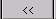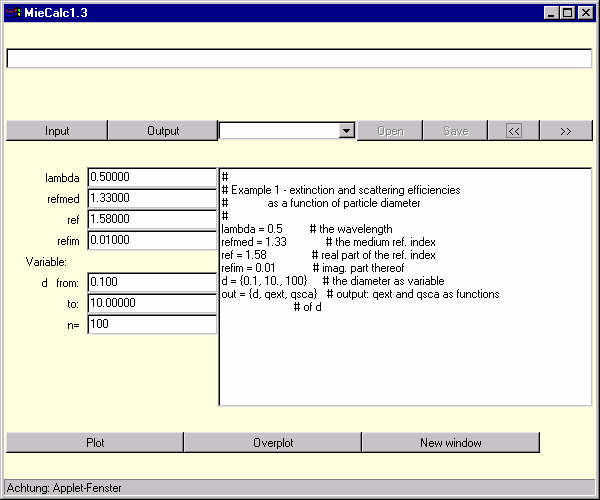### Using the Program's Editor

Instead of using the input/output dialogs to specify input and output parameters, you may also use the text area on the right side of the program window.  Clear the editor's text area by selecting the empty item in the select-box (see functional elements of the main window) For the text, the following syntax rules apply:
1. Constants are entered in the form   param = value  where   param is the name of the parameter and value its numerical value. Examples:   lambda=0.6328, refmed=1.0, ref = 1.4,   etc.
2. Variables are specified in the form    variable = {lowerbound, upperbound, n},  where lowerbound is the lower bound of its range of variation, upperbound is the upper bound and n is the number of data points. Example:   d= {0.02, 20., 200}
3. Tabulated parameters are specified in the form   in = {param1, param2, param3, ....}. This means that param1 is read in from column 1 of the table, param2 from column 2 etc. Use the keyword null to skip a column. For example write   in= {param1, null, param2} to skip the second column of the table. See the description of tables below.
4. Output parameters are specified as follows:     out = {abscissa, ordinate1, ordinate2, ....},    where abscissa is the parameter to be plotted along the abscissa, and ordinate1, ordinate2, etc are the ordinates.  Example: write out = {d, Qext, Qsca} to plot the extinction and scattering efficiences as functions of the diameter.
5. Empty lines and all text following a #  are regarded as comments.
6. The table containing the tabulated parameters must follow after the parameter definitions.
In order to reproduce the result of the previous section, enter the following text into the editor:

```#
# Example 1 - extinction and scattering efficiencies
#             as a function of particle diameter
#
lambda = 0.5         # the wavelength
refmed = 1.33             # the medium ref. index
ref = 1.58               # real part of the ref. index
refim = 0.01            # imag. part thereof
d = {0.1, 10., 100}     # the diameter as variable
out = {d, qext, qsca}   # output: qext and qsca as functions
# of d```
After entering the text, click on. The text in the text area is then interpreted and the input/output parameters are set correspondingly:You may edit these settings by use of the input/output dialog boxes or by changing the numerical values in the number fields on the left side of the program window. After editing, proceed as before, clicking on the PLOT, OVERPLOT, or NEW WINDOW buttons. The use of the text area is mainly to read data from file or databases - see below.